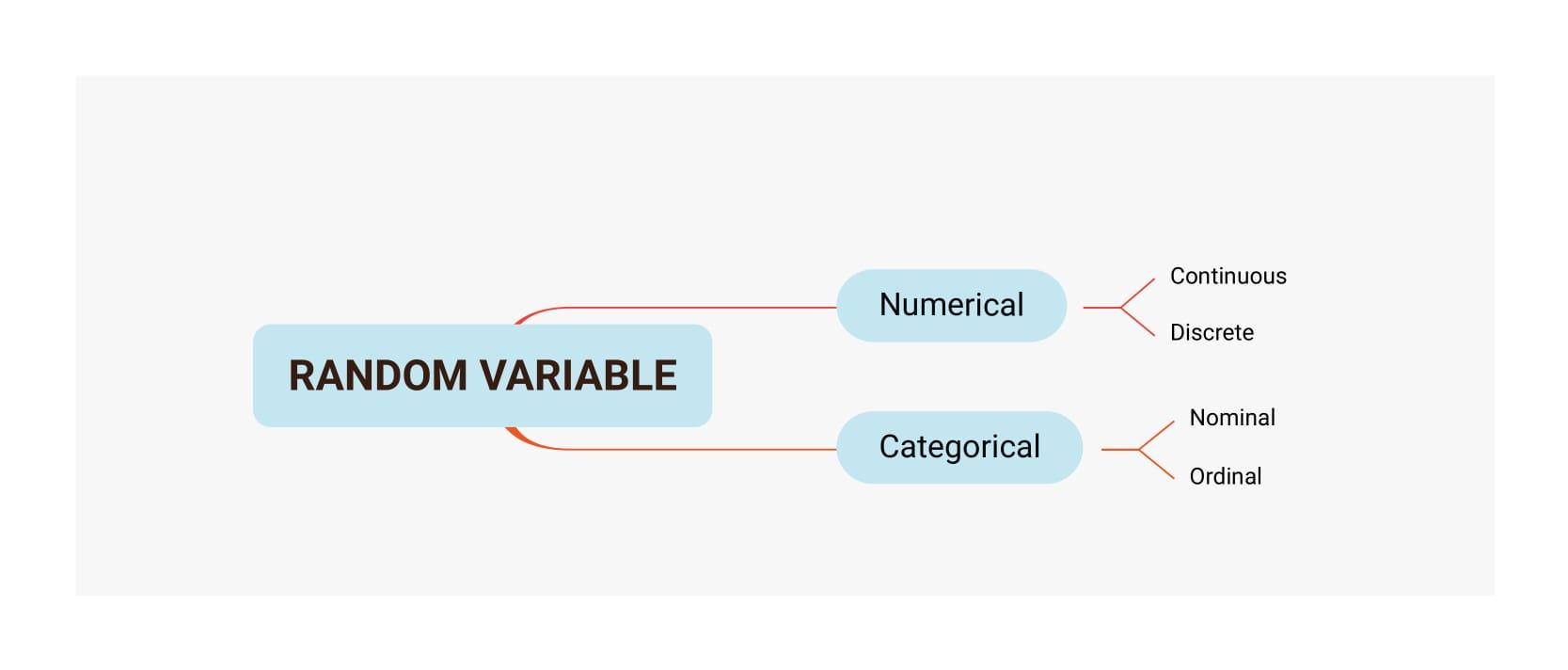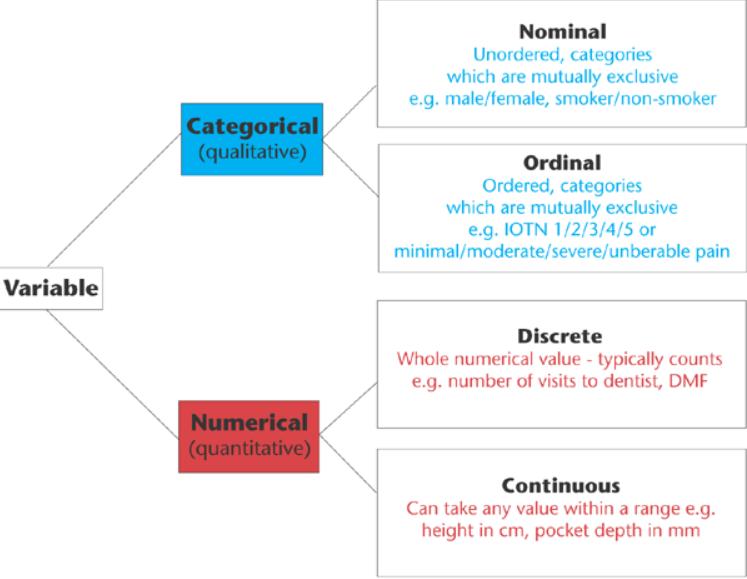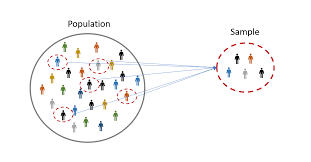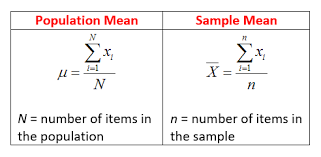# STATISTICS FOR DATA SCIENCE## 2. Random variable : It is a random collection of variables.

A. Numerical variable : A numerical is one that may take on any value within a finite or infinite interval (e.g., height, weight, temperature, blood glucose, …)

Numerical variable is further divided into two parts :

A.1. Continuous(floating number) : A continuous variable is one which have decimal values. For example : 5.6, 7.8, 0.001, 846.245

A.2. Discrete(whole number) : Discrete numbers are the basic counting numbers. For example : 0, 1, 2, 3, 4, 5, 6

B. Categorical Variable : A categorical variable is a variable that can take on one of a limited, and usually fixed, number of possible values (e.g. race, sex, age group)

Categorical Variable is further divided into two parts :

B.1. Nominal : A nominal variable does not have orders.

B.2. Ordinal : An ordinal variable is a categorical variable for which the possible values are ordered (e.g. education level (“high school”, ”BS”, ”MS”, ”PhD”))

## RANDOM VARIABLE CONCLUSION :3. MEASURE OF CENTRAL TENDENCIES :

A. MEAN : it is the sum of a collection of numbers divided by the count of numbers in the collection

mean = sum of number of collection / total collection

B. MEDIAN : The “middle” of a sorted list of numbers(When there are two middle numbers we average them).

C. MODE : The mode of a set of data values is the value that appears most often.

NOTE : mean, median, mode helps in handling missing values.

4. RANGE : The Range is the difference between the lowest and highest values. Example: In {4, 6, 9, 3, 7} the lowest value is 3, and the highest is 9. So the range is 9 − 3 = 6.

5. POPULATION, SAMPLE, POPULATION MEAN, SAMPLE MEAN :

POPULATION : a population is a set of similar items or events.

SAMPLE : small collection of items from population.

Every dataset that we get to perform ML model is a sample of data.

Population vs sample use case : exit poll on election.

POPULATION MEAN : The population mean is an average of a group characteristic.

SAMPLE MEAN : A sample mean refers to the average of the sample data.POPULATION VS SAMPLEPOPULATION MEAN VS SAMPLE MEAN 6. VARIANCE : variance : It is the desire for the squared deviation of a random variable from its mean. Informally, it measures how far a set of numbers is spread out from their average worth. 7. Standard deviation and measure of dispersion: Standard deviation (SD) is the most usually utilized measure of dispersion. It is a measure of spread of data about the mean. SD is the square root of aggregate of squared deviation from the mean isolated by the number of observations.The standard deviation is a measure of the measure of variation or dispersion of a set of values. A low standard deviation shows that the values will in general be near the mean of the set, while an exclusive requirement deviation demonstrates that the values are spread out over a wider range.                                 STANDARD DEVIATION